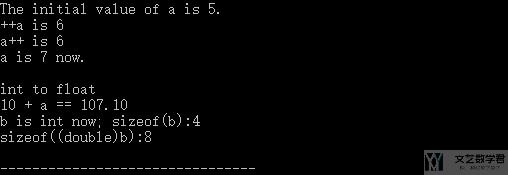# C学习笔记 – 数据类型转换和运算符

• A+

## 内容概括

• 强制类型转换 : (double)a //将a转换为double形式 ；
• 自增自减运算符 ++i :   --i（在使用 i 之前，先使 i 加（减）1 ）,先执行i=i+1, 再使用i的值 ;
• 自增运算符 i++ : i--（在使用 i 之后，使 i 的值加（减）1 ）;

## 源代码

1. #include<stdio.h>
2. /*
3.     这一部分是关于运算符和数据类型转换的讲解
4. */
5. int main(){
6.     /*
7.     自增自减运算符
8.     1. ++i ，--i（在使用 i 之前，先使 i 加（减）1 ）,先执行i=i+1, 再使用i的值 ;
9.     2. i++，i--（在使用 i 之后，使 i 的值加（减）1 ）;
10.     */
11.     int a = 5;
12.     printf("The initial value of a is %d.\n",a);
13.     printf("++a is %d\n",++a);
14.     // >> ++a is 6
15.     printf("a++ is %d\n",a++);
16.     // >> a++ is 6
17.     printf("a is %d now.\n",a);
18.     // >> a is 7 now
19.     // ---------------------
20.     // ---------------------
21.     // ---------------------
22.     /*
23.     不同数据类型之间的混合运算
24.     1. 会往数据类型优先级高的转换,如int和float运算,会先把int转为float;
25.     2. char与int进行运算时,就是ASCII与int运算;
26.     */
27.     printf("\nint to float\n");
28.     printf("10 + a == %0.2f\n",10.1+'a');
29.     // >> 10 + a == 107.10
30.     /*
31.     强制类型转换
32.     (double)a //将a转换为double形式
33.     */
34.     int b = 1;
35.     printf("b is int now; sizeof(b):%d\n",sizeof(b));
36.     // >> sizeof(b):4
37.     printf("sizeof((double)b):%d\n",sizeof((double)b));
38.     // >> sizeof((double)b):8
39.     return 0;
40. }• 微信公众号
• 关注微信公众号
•• QQ群
• 我们的QQ群号
•